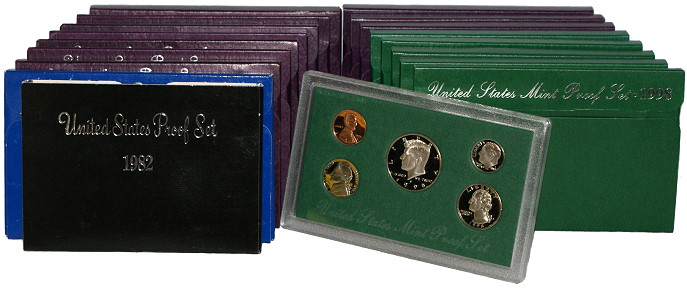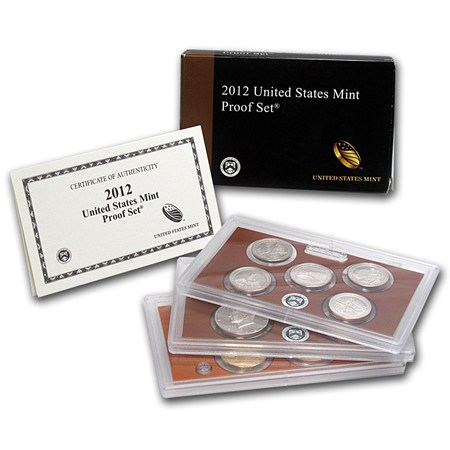# Buying List: U.S. Mint Proof and Uncirculated Mint Sets## US MINT Proof and Mint Set BID SHEET

### Here is a partial list of the sets we buy with current bids. PRICES ARE FOR EXCELLENT, UNFLAWED COINS WITHOUT BLEMISHES, CLOUDINESS, OR TONING and CLEAN UNDAMAGED BOXES!

Year Description We Pay
1936-1949 Proof Sets inquire
1950 Proof Set (tan box, 5 coins) we pay \$200.00
1951 Proof Set (tan box, 5 coins) we pay \$125.00
1952 Proof Set (tan box, 5 coins) we pay \$60.00
1953 Proof Set (tan box, 5 coins) we pay \$50.00
1954 Proof Set (tan box, 5 coins) we pay \$30.00
1955 Proof Set (tan box, 5 coins) we pay \$30.00
1956 Proof Set (tan envelope, 5 coins) we pay \$30.00
1957 Proof Set (tan envelope, 5 coins) we pay \$25.00
1958 Proof Set (tan envelope, 5 coins) we pay \$15.00
1959 Proof Set (tan envelope, 5 coins) we pay \$15.00
1960 Proof Set (tan envelope, 5 coins) we pay \$15.00
1960 Uncirculated Set (white envelope, 10 coins) we pay \$20.00
1961 Proof Set (tan envelope, 5 coins) we pay \$10.00
1961 Uncirculated Set (white envelope, 10 coins) we pay \$20.00
1962 Proof Set (tan envelope, 5 coins) we pay \$10.00
1962 Uncirculated Set (white envelope, 10 coins) we pay \$20.00
1963 Proof Set (tan envelope, 5 coins) we pay \$10.00
1963 Uncirculated Set (white envelope, 10 coins) we pay \$20.00
1964 Proof Set (tan envelope, 5 coins) we pay \$10.00
1964 Uncirculated Set (white envelope, 10 coins) we pay \$20.00
1965 Special Mint Set (white envelope, 5 coins) we pay \$5.00
1966 Special Mint Set (5 coins) we pay \$4.00
1967 Special Mint Set (blue box, 5 coins) we pay \$4.00
1968 Proof Set (blue box, 5 coins) we pay \$4.00
1968 Uncirculated Set (white envelope, 10 coins) we pay \$6.00
1969 Proof Set (blue box, 5 coins) we pay \$3.00
1969 Uncirculated Set (white envelope, 10 coins) we pay \$5.00
1970 Proof Set (blue box, 5 coins) we pay \$3.00
1970 Uncirculated Set (white envelope, 10 coins) we pay \$6.00
1971 Proof Set (blue box, 5 coins) we pay \$3.00
1971 Uncirculated Set (white envelope, 10 coins) we pay \$6.00
1972 Proof Set (blue box, 5 coins) we pay \$3.00
1972 Uncirculated Set (white envelope, 10 coins) we pay \$4.00
1973 Proof Set (black box, 5 coins) we pay \$2.00
1973 Uncirculated Set (white envelope, 10 coins) we pay \$4.00
1974 Proof Set (black box, 5 coins) we pay \$2.00
1974 Uncirculated Set (white envelope, 10 coins) we pay \$3.00
1975 Proof Set (black box, 5 coins) we pay \$2.00
1976 Proof Set (black box, 6 coins) we pay \$2.00
1976 Uncirculated Set (white envelope, 12 coins) we pay \$4.00
1976 Silver Proof Set - 3 coins - dark blue folder we pay \$10.00
1976 Silver Uncirculated Set - 3 coins - red envelope we pay \$6.00
1977 Proof Set (black box, 6 coins) we pay \$3.00
1977 Uncirculated Set (white envelope, 12 coins) we pay \$4.00
1978 Proof Set (black box, 6 coins) we pay \$3.00
1979 Proof Set (black box, 6 coins) we pay \$3.00
1980 Proof Set (black box, 6 coins) we pay \$3.00
1981 Proof Set (black box, 6 coins) we pay \$3.00
1982 Proof Set (black box, 5 coins) we pay \$2.00
1983 Proof Set (blue box, 5 coins) we pay \$2.00
1984 Proof Set (purple box, 5 coins) we pay \$2.00
1985 Proof Set (purple box, 5 coins) we pay \$2.00
1986 Proof Set (purple box, 5 coins) we pay \$2.00
1987 Proof Set (purple box, 5 coins) we pay \$2.00
1988 Proof Set (purple box, 5 coins) we pay \$2.00
1989 Proof Set (purple box, 5 coins) we pay \$2.00
1990 Proof Set (purple box, 5 coins) we pay \$2.00
1990 Prestige Proof Set (green box, 6 coins, with certificate intact) we pay \$10.00
1991 Proof Set (purple box, 5 coins) we pay \$2.00
1992 Proof Set (purple box, 5 coins) we pay \$2.00
1992 Silver Proof Set (black box, 5 coins) we pay \$10.00
1993 Proof Set (purple box, 5 coins) we pay \$2.00
1993 Silver Proof Set (black box, 5 coins) we pay \$10.00
1994 Proof Set (green box, 5 coins) we pay \$2.00
1994 Silver Proof Set (black box, 5 coins) we pay \$10.00
1995 Proof Set (green box, 5 coins) we pay \$2.00
1995 Silver Proof Set (black box, 5 coins) we pay \$10.00
1996 Proof Set (green box, 5 coins) we pay \$6.00
1996 Silver Proof Set (black box, 5 coins) we pay \$10.00
1996 Uncirculated Set (brown envelope, 11 coins including loose dime) we pay \$10.00
1997 Proof Set (5 coins, green box) we pay \$2.00
1997 Silver Proof Set (black box, 5 coins) we pay \$10.00
1998 Proof Set (5 coins, green box) we pay \$2.00
1998 Silver Proof Set (black box, 5 coins) we pay \$10.00
1999 Proof Set (blue box, 9 coins) we pay \$6.00
1999 Silver Proof Set (red box, 9 coins) we pay \$45.00
2000 Proof Set (blue box, 10 coins) we pay \$5.00
2000 Silver Proof Set (red box, 10 coins) we pay \$15.00
2000 Uncirculated Coin Set (red and blue envelopes, 20 coins) we pay \$4.00
2001 Proof Set (blue box, 10 coins) we pay \$5.00
2001 Silver Proof Set (red box, 10 coins) we pay \$15.00
2001 Uncirculated Coin Set (red and blue envelopes, 20 coins) we pay \$6.00
2002 Proof Set (blue box, 10 coins) we pay \$5.00
2002 Silver Proof Set (red box, 10 coins) we pay \$15.00
2002 Uncirculated Coin Set (red and blue envelopes, 20 coins) we pay \$6.00
2003 Proof Set (blue box, 10 coins) we pay \$5.00
2003 Silver Proof Set (red box, 10 coins) we pay \$15.00
2003 Uncirculated Coin Set (red and blue envelopes, 20 coins) we pay \$6.00
2004 Proof Set (blue box, 11 coins) we pay \$5.00
2004 Silver Proof Set (red box, 11 coins) we pay \$15.00
2004 50 State Quarters Proof Set (blue box, 5 coins) we pay \$1.50
2004 50 State Quarters Silver Proof Set (black/white box, 5 coins) we pay \$6.00
2004 Uncirculated Coin Set (red and blue envelopes, 20 coins) we pay \$6.00
2005 Proof Set (blue box, 11 coins) we pay \$5.00
2005 Silver Proof Set (red box, 11 coins) we pay \$15.00
2005 50 State Quarters Proof Set (blue box, 5 coins) we pay \$1.50
2005 50 State Quarters Silver Proof Set (black/white box, 5 coins) we pay \$6.00
2005 American Legacy Collection Silver Proof Set (black box, 13 coins) we pay \$25.00
2005 Uncirculated Coin Set (red and blue envelopes, 22 coins) we pay \$6.00
2006 Proof Set (blue box, 10 coins) we pay \$5.00
2006 Silver Proof Set (red box, 10 coins) we pay \$15.00
2006 50 State Quarters Proof Set (blue box, 5 coins) we pay \$1.50
2006 50 State Quarters Silver Proof Set (gray box, 5 coins) we pay \$8.00
2006 Uncirculated Coin Set (red and blue envelopes, 20 coins) we pay \$4.00
2007 Proof Set (blue box, 14 coins) we pay \$5.00
2007 Silver Proof Set (red box, 14 coins) we pay \$15.00
2007 50 State Quarters Proof Set (blue box, 5 coins) we pay \$1.50
2007 50 State Quarters Silver Proof Set (black/white box, 5 coins) we pay \$6.00
2007 Uncirculated Coin Set (red and blue envelopes, 28 coins) we pay \$7.00
2008 Proof Set (blue/white box, 14 coins) we pay \$10.00
2008 Silver Proof Set (red box, 14 coins) we pay \$18.00
2008 50 State Quarters Proof Set (blue box, 5 coins) we pay \$1.50
2008 50 State Quarters Silver Proof Set (black/white box, 5 coins) we pay \$6.00
2008 Uncirculated Coin Set (red and blue envelopes, 28 coins) we pay \$7.00
2008 Annual Uncirculated Dollar Coin Set (red, white and blue folder, 6 coins) we pay \$22.00
2009 Proof Set (light blue box, 18 coins) we pay \$10.00
2009 Silver Proof Set (red box, 18 coins) we pay \$18.00
2009 Uncirculated Coin Set (brown and blue folders, 36 coins) we pay \$15.00
2009 Annual Uncirculated Dollar Coin Set (red, white and blue folder, 6 coins) we pay \$22.00
2010 Proof Set (light blue box, 14 coins) we pay \$10.00
2010 Presidential Dollar Proof Set (4 coins) we pay \$3.00
2010 America The Beautiful Proof Set (5 coins) we pay \$3.00
2010 Silver Proof Set (red box, 14 coins) we pay \$15.00
2010 Uncirculated Coin Set (brown and blue folders, 28 coins) we pay \$9.00
2010 Annual Uncirculated Dollar Coin Set (red, white and blue folder, 6 coins) we pay \$22.00
2011 Proof Set (black/gold box, 14 coins) we pay \$10.00
2011 Silver Proof Set (black/silver box, 14 coins) we pay \$20.00
2011 Uncirculated Coin Set (black folders, Denver and Philadelphia, 28 coins) we pay \$9.00
2011 Annual Uncirculated Dollar Coin Set (red, white and blue folder, 6 coins) we pay \$22.00
2012 Proof Set (black/gold box, 14 coins) we pay \$50.00
2012 Silver Proof Set (black/silver box, 14 coins) we pay \$120.00
2012 Uncirculated Coin Set (black folders, Denver and Philadelphia, 28 coins) we pay \$15.00
2012 Annual Uncirculated Dollar Coin Set (red, white and blue folder, 6 coins) we pay \$22.00
2013 Proof Set (14 coins) we pay \$7.00
2013 Silver Proof Set (14 coins) we pay \$25.00
2013 Annual Uncirculated Dollar Coin Set (red, white and blue folder, 6 coins) we pay \$22.00
2013 Uncirculated Coin Set (black folders, Denver and Philadelphia, 28 coins) we pay \$9.00
2014 Proof Set (14 coins) we pay \$10.00
2013 Silver Proof Set (14 coins) we pay \$25.00
2014 Uncirculated Coin Set (black folders, Denver and Philadelphia, 28 coins) we pay \$9.00
2014 Annual Uncirculated Dollar Coin Set (red, white and blue folder, 6 coins) we pay \$22.00
2015 Proof Set (14 coins) we pay \$15.00
2013 Silver Proof Set (14 coins) we pay \$25.00
2015 Uncirculated Coin Set (black folders, Denver and Philadelphia, 28 coins) we pay \$9.00
2015 Annual Uncirculated Dollar Coin Set (red, white and blue folder, 6 coins) we pay \$22.00
2016 Proof Set (13 coins) we pay \$15.00
2016 Silver Proof Set (13 coins) we pay \$35.00
2016 Uncirculated Coin Set (black folders, Denver and Philadelphia, 28 coins) we pay \$15.00
2016 Annual Uncirculated Dollar Coin Set (red, white and blue folder, 6 coins) we pay \$22.00
2017 Proof Set (10 coins) we pay \$15.00
2017 Silver Proof Set (10 coins) we pay \$25.00
2017 Uncirculated Coin Set (black folders with American Flag, Denver and Philadelphia, 28 coins) we pay \$15.00
2017 Annual Uncirculated Dollar Coin Set (red, white and blue folder, 6 coins) we pay \$22.00
2018 Happy Birthday Set (5 coins) we pay \$15.00
2018 Proof Set (10 coins) we pay \$15.00
2018 Silver Proof Set (10 coins) we pay \$30.00
2018 Uncirculated Set (20 coins) we pay \$15.00
2018 Annual Uncirculated Dollar Coin Set (red, white and blue folder, 6 coins) we pay \$22.00
2018 Reverse Silver Proof Set (10 coins) we pay \$50.00
2018 Limited Edition Silver Proof Set (black box, 10 coins) we pay \$110.00
2019 Proof Set (10 coins) we pay \$7.00
2019 Silver Proof Set (10 coins) we pay \$30.00
2019 Uncirculated Set (20 coins) we pay \$15.00
2019 Annual Uncirculated Dollar Coin Set (red, white and blue folder, 6 coins) we pay \$22.00
2020 Annual Uncirculated Dollar Coin Set (red, white and blue folder, 6 coins) we pay \$22.00
2020 Proof Set (10 coins) we pay \$25.00
2020 Silver Proof Set (10 coins) we pay \$70.00

## What is a proof set worth?

### Proof sets can be worth lots of money, such as this 2012 US Mint set. If you have US proof sets in top condition, contact us.